The MEANS Procedure

# PROC MEANS Statement

 See also: The SUMMARY Procedure

 PROC MEANS ;

Task Option
Specify the input data set DATA=
Disable floating point exception recovery NOTRAP
Specify the amount of memory to use for data summarization with class variables SUMSIZE=
Override the SAS system option THREADS | NOTHREADS THREADS | NOTHREADS
Control the classification levels

Specify a secondary data set that contains the combinations of class variables to analyze CLASSDATA=

Create all possible combinations of class variable values COMPLETETYPES

Exclude from the analysis all combinations of class variable values that are not in the CLASSDATA= data set EXCLUSIVE

Use missing values as valid values to create combinations of class variables MISSING
Control the statistical analysis

Specify the confidence level for the confidence limits ALPHA=

Exclude observations with nonpositive weights from the analysis EXCLNPWGT

Specify the sample size to use for the P2 quantile estimation method QMARKERS=

Specify the quantile estimation method QMETHOD=

Specify the mathematical definition used to compute quantiles QNTLDEF=

Select the statistics statistic-keyword

Specify the variance divisor VARDEF=
Control the output

Specify the field width for the statistics FW=

Specify the number of decimal places for the statistics MAXDEC=

Suppress reporting the total number of observations for each unique combination of the class variables NONOBS

Suppress all displayed output NOPRINT

Order the values of the class variables according to the specified order ORDER=

Display the output PRINT

Display the analysis for all requested combinations of class variables PRINTALLTYPES

Display the values of the ID variables PRINTIDVARS
Control the output data set

Specify that the _TYPE_ variable contain character values. CHARTYPE

Order the output data set by descending _TYPE_ value DESCENDTYPES

Select ID variables based on minimum values IDMIN

Limit the output statistics to the observations with the highest _TYPE_ value NWAY

ALPHA=value

specifies the confidence level to compute the confidence limits for the mean. The percentage for the confidence limits is (1-value)×100. For example, ALPHA=.05 results in a 95% confidence limit.

 Default: .05 Range: between 0 and 1 Interaction: To compute confidence limits specify the statistic-keyword CLM, LCLM, or UCLM. See also: Confidence Limits Featured in: Computing a Confidence Limit for the Mean
CHARTYPE

specifies that the _TYPE_ variable in the output data set is a character representation of the binary value of _TYPE_. The length of the variable equals the number of class variables.

 Interaction: When you specify more than 32 class variables, _TYPE_ automatically becomes a character variable. Main discussion: Output Data Set Featured in: Computing Output Statistics with Missing Class Variable Values
CLASSDATA=SAS-data-set

specifies a data set that contains the combinations of values of the class variables that must be present in the output. Any combinations of values of the class variables that occur in the CLASSDATA= data set but not in the input data set appear in the output and have a frequency of zero.

 Restriction: The CLASSDATA= data set must contain all class variables. Their data type and format must match the corresponding class variables in the input data set. Interaction: If you use the EXCLUSIVE option, then PROC MEANS excludes any observation in the input data set whose combination of class variables is not in the CLASSDATA= data set. Tip: Use the CLASSDATA= data set to filter or to supplement the input data set. Featured in: Using a CLASSDATA= Data Set with Class Variables
COMPLETETYPES

creates all possible combinations of class variables even if the combination does not occur in the input data set.

 Interaction: The PRELOADFMT option in the CLASS statement ensures that PROC MEANS writes all user-defined format ranges or values for the combinations of class variables to the output, even when a frequency is zero. Tip: Using COMPLETETYPES does not increase the memory requirements. Featured in: Using Preloaded Formats with Class Variables
DATA=SAS-data-set

identifies the input SAS data set.

 Main discussion: Input Data Sets
DESCENDTYPES

orders observations in the output data set by descending _TYPE_ value.

 Alias: DESCENDING | DESCEND Interaction: Descending has no effect if you specify NWAY. Tip: Use DESCENDTYPES to make the overall total (_TYPE_=0) the last observation in each BY group. See also: Output Data Set Featured in: Computing Different Output Statistics for Several Variables
EXCLNPWGT

excludes observations with nonpositive weight values (zero or negative) from the analysis. By default, PROC MEANS treats observations with negative weights like observations with zero weights and counts them in the total number of observations.

 Alias: EXCLNPWGTS See also: WEIGHT= and WEIGHT Statement
EXCLUSIVE

excludes from the analysis all combinations of the class variables that are not found in the CLASSDATA= data set.

 Requirement: If a CLASSDATA= data set is not specified, then this option is ignored. Featured in: Using a CLASSDATA= Data Set with Class Variables
FW=field-width

specifies the field width to display the statistics in printed or displayed output. FW= has no effect on statistics that are saved in an output data set.

 Default: 12 Tip: If PROC MEANS truncates column labels in the output, then increase the field width. Featured in:
IDMIN

specifies that the output data set contain the minimum value of the ID variables.

 Interaction: Specify PRINTIDVARS to display the value of the ID variables in the output. See also: ID Statement
MAXDEC=number

specifies the maximum number of decimal places to display the statistics in the printed or displayed output. MAXDEC= has no effect on statistics that are saved in an output data set.

 Default: BEST. width for columnar format, typically about 7. Range: 0-8 Featured in:
MISSING

considers missing values as valid values to create the combinations of class variables. Special missing values that represent numeric values (the letters A through Z and the underscore (_) character) are each considered as a separate value.

 Default: If you omit MISSING, then PROC MEANS excludes the observations with a missing class variable value from the analysis. See also: SAS Language Reference: Concepts for a discussion of missing values that have special meaning. Featured in: Using Preloaded Formats with Class Variables
NONOBS

suppresses the column that displays the total number of observations for each unique combination of the values of the class variables. This column corresponds to the _FREQ_ variable in the output data set.

 See also: The N Obs Statistic Featured in:
NOPRINT

See PRINT | NOPRINT.

NOTHREADS
NOTRAP

disables floating point exception (FPE) recovery during data processing. By default, PROC MEANS traps these errors and sets the statistic to missing.

In operating environments where the overhead of FPE recovery is significant, NOTRAP can improve performance. Note that normal SAS FPE handling is still in effect so that PROC MEANS terminates in the case of math exceptions.

NWAY

specifies that the output data set contain only statistics for the observations with the highest _TYPE_ and _WAY_ values. When you specify class variables, NWAY corresponds to the combination of all class variables.

 Interaction: If you specify a TYPES statement or a WAYS statement, then PROC MEANS ignores this option. See also: Output Data Set Featured in: Computing Output Statistics with Missing Class Variable Values
ORDER=DATA | FORMATTED | FREQ | UNFORMATTED

specifies the sort order to create the unique combinations for the values of the class variables in the output, where

DATA

orders values according to their order in the input data set.

 Interaction: If you use PRELOADFMT in the CLASS statement, then the order for the values of each class variable matches the order that PROC FORMAT uses to store the values of the associated user-defined format. If you use the CLASSDATA= option, then PROC MEANS uses the order of the unique values of each class variable in the CLASSDATA= data set to order the output levels. If you use both options, then PROC MEANS first uses the user-defined formats to order the output. If you omit EXCLUSIVE, then PROC MEANS appends after the user-defined format and the CLASSDATA= values the unique values of the class variables in the input data set based on the order in which they are encountered. Tip: By default, PROC FORMAT stores a format definition in sorted order. Use the NOTSORTED option to store the values or ranges of a user defined format in the order that you define them.
FORMATTED

orders values by their ascending formatted values. This order depends on your operating environment.

 Alias: FMT | EXTERNAL
FREQ

orders values by descending frequency count so that levels with the most observations are listed first.

 Interaction: For multiway combinations of the class variables, PROC MEANS determines the order of a class variable combination from the individual class variable frequencies. Interaction: Use the ASCENDING option in the CLASS statement to order values by ascending frequency count.
UNFORMATTED

orders values by their unformatted values, which yields the same order as PROC SORT. This order depends on your operating environment.

 Alias: UNFMT | INTERNAL Default: UNFORMATTED See also: Ordering the Class Values
PCTLDEF=

PCTLDEF is an alias for QNTLDEF=.

 See also: QNTLDEF=
PRINT | NOPRINT

specifies whether PROC MEANS displays the statistical analysis. NOPRINT suppresses all the output.

 Default: PRINT Tip: Use NOPRINT when you want to create only an OUT= output data set. Featured in: For an example of NOPRINT, see
PRINTALLTYPES

displays all requested combinations of class variables (all _TYPE_ values) in the printed or displayed output. Normally, PROC MEANS shows only the NWAY type.

 Alias: PRINTALL Interaction: If you use the NWAY option, the TYPES statement, or the WAYS statement, then PROC MEANS ignores this option. Featured in: Using a CLASSDATA= Data Set with Class Variables
PRINTIDVARS

displays the values of the ID variables in printed or displayed output.

 Alias: PRINTIDS Interaction: Specify IDMIN to display the minimum value of the ID variables. See also: ID Statement
QMARKERS=number

specifies the default number of markers to use for the P² quantile estimation method. The number of markers controls the size of fixed memory space.

 Default: The default value depends on which quantiles you request. For the median (P50), number is 7. For the quantiles (P25 and P50), number is 25. For the quantiles P1, P5, P10, P75 P90, P95, or P99, number is 105. If you request several quantiles, then PROC MEANS uses the largest value of number. Range: an odd integer greater than 3 Tip: Increase the number of markers above the defaults settings to improve the accuracy of the estimate; reduce the number of markers to conserve memory and computing time. Main Discussion: Quantiles
QMETHOD=OS|P2|HIST

specifies the method that PROC MEANS uses to process the input data when it computes quantiles. If the number of observations is less than or equal to the QMARKERS= value and QNTLDEF=5, then both methods produce the same results.

OS

uses order statistics. This method is the same method that PROC UNIVARIATE uses.

Note:   This technique can be very memory-intensive.P2|HIST

uses the P² method to approximate the quantile.

 Default: OS Restriction: When QMETHOD=P2, PROC MEANS will not compute the following: MODE weighted quantiles Tip: When QMETHOD=P2, reliable estimations of some quantiles (P1,P5,P95,P99) might not be possible for some data sets. Main Discussion: Quantiles
QNTLDEF=1|2|3|4|5

specifies the mathematical definition that PROC MEANS uses to calculate quantiles when QMETHOD=OS. To use QMETHOD=P2, you must use QNTLDEF=5.

 Alias: PCTLDEF= Default: 5 Main discussion: Quantile and Related Statistics
statistic-keyword(s)

specifies which statistics to compute and the order to display them in the output. The available keywords in the PROC statement are

 Descriptive statistic keywords CLM NMISS CSS RANGE CV SKEWNESS|SKEW KURTOSIS|KURT STDDEV|STD LCLM STDERR MAX SUM MEAN SUMWGT MIN UCLM MODE USS N VAR Quantile statistic keywords MEDIAN|P50 Q3|P75 P1 P90 P5 P95 P10 P99 Q1|P25 QRANGE Hypothesis testing keywords PROBT|PRT T

 Default: N, MEAN, STD, MIN, and MAX Requirement: To compute standard error, confidence limits for the mean, and the Student's t-test, you must use the default value of the VARDEF= option, which is DF. To compute skewness or kurtosis, you must use VARDEF=N or VARDEF=DF. Tip: Use CLM or both LCLM and UCLM to compute a two-sided confidence limit for the mean. Use only LCLM or UCLM, to compute a one-sided confidence limit. Main discussion: The definitions of the keywords and the formulas for the associated statistics are listed in Keywords and Formulas. Featured in:
SUMSIZE=value

specifies the amount of memory that is available for data summarization when you use class variables. value might be one of the following:

n|nK| nM| nG

specifies the amount of memory available in bytes, kilobytes, megabytes, or gigabytes, respectively. If n is 0, then PROC MEANS use the value of the SAS system option SUMSIZE=.

MAXIMUM|MAX

specifies the maximum amount of memory that is available.

 Default: The value of the SUMSIZE= system option. Tip: For best results, do not make SUMSIZE= larger than the amount of physical memory that is available for the PROC step. If additional space is needed, then PROC MEANS uses utility files. Main discussion: Computational Resources Note:   Specifying SUMSIZE=0 enables proc MEANS to use the preferred global REALMEMSIZE option.See also: The SAS system option SUMSIZE= in SAS Language Reference: Dictionary.
THREADS | NOTHREADS

enables or disables parallel processing of the input data set. This option overrides the SAS system option THREADS | NOTHREADS unless the system option is restricted (see Restriction). See SAS Language Reference: Concepts for more information about parallel processing.

 Default: value of SAS system option THREADS | NOTHREADS. Restriction: Your site administrator can create a restricted options table. A restricted options table specifies SAS system option values that are established at startup and cannot be overridden. If the THREADS | NOTHREADS system option is listed in the restricted options table, any attempt to set these system options is ignored and a warning message is written to the SAS log. Interaction: PROC MEANS honors the SAS system option THREADS except when a BY statement is specified or the value of the SAS system option CPUCOUNT is less than 2. You can use THREADS in the PROC MEANS statement to force PROC MEANS to use parallel processing in these situations.

Note:   If THREADS is specified (either as a SAS system option or on the PROC MEANS statement) and another program has the input data set open for reading, writing, or updating, then PROC MEANS might fail to open the input data set. In this case, PROC MEANS stops processing and writes a message to the SAS log.VARDEF=divisor

specifies the divisor to use in the calculation of the variance and standard deviation. The following table shows the possible values for divisor and associated divisors.

Possible Values for VARDEF=
Value Divisor Formula for Divisor
DF degrees of freedom n - 1
N number of observations n
WDF sum of weights minus one (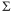iwi) - 1
WEIGHT | WGT sum of weightsiwi

The procedure computes the variance as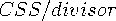, where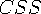is the corrected sums of squares and equals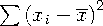. When you weight the analysis variables,equals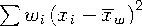, where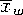is the weighted mean.

 Default: DF Requirement: To compute the standard error of the mean, confidence limits for the mean, or the Student's t-test, use the default value of VARDEF=. Tip: When you use the WEIGHT statement and VARDEF=DF, the variance is an estimate of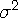, where the variance of the ith observation is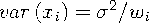and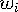is the weight for the ith observation. This method yields an estimate of the variance of an observation with unit weight. Tip: When you use the WEIGHT statement and VARDEF=WGT, the computed variance is asymptotically (for large n) an estimate of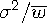, where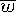is the average weight. This method yields an asymptotic estimate of the variance of an observation with average weight. Main discussion: Keywords and Formulas See also: Weighted Statistics ExamplePrevious Page | Next Page | Top of Page